Do you like the article?
Share it with others -
Use new possibilities of MetaTrader 5

# 20 Trade Signals in MQL5

26 August 2010, 16:59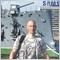8
23 263

### Introduction

Traders try to find regularities in the price behavior, strive to form rules using which they will have a good chance to determine a favorable moment for buying or selling. To create a fully automatic system, you need to learn how to inform about the forthcoming of such moments - trade signals.

Signals inform traders about the potential points of entering a position, however not all of them are obligatory to execute. Additional criteria can filter out even most of the signals, but it's not significant for us. The subject of the article is how to program the most popular trade signals in MQL5.

### 1. What Signals Do We Know?

All methods of determining the moments of entering the market can be divided into several types:

• intersection of moving averages
• breakthrough of a range
• exit from the zone of overbuying/overselling on the basis of stochastic rates
• bounce from borders of a channel
• breakthrough of borders of a channel
• change of a trend

### 2. How to Code It Better?

There are a lot of ways of writing a code; it can be written within the OnStart(), OnTick() and the other functions. You can find a more detailed information about them in the documentation at this website or in the user guide embedded in the MetaEditor; however this method is not effective and sometimes you need to write the same parts of the code for several times. That's way we'll take another way - we will use custom functions that can be called from any part of the code of our program.

For convenience of using the created functions, let's combine them in a separate group as an external include file and name it SignalTrade; it will be stored in the ...\MQL5\Include directory. It will allow connecting this module easily to any programs.

Despite all the signals look different, they have many things in common. There are three variants that can be received from a function that generates the signals:

• signal to sell
• no signal

So let's create an enumeration that corresponds to these signals.

•  1 - signal to buy
• -1 - signal to sell
•  0 - no signal

Let's write a prototype of the function that returns a signal. Divide our function into several parts where one or another operation will be performed. Variables necessary for storing data are declared and initialized at the beginning of the function. The loading and checking of necessary information from the created indicator will be performed further. Checking of information is essential to avoid unforeseen consequences of working with data and of the program in whole.

Once the information is loaded and checked, go to forming a signal. As soon as a signal is formed, exit the function and return the obtained signal to it as one of the values mentioned above.

```int TradeSignal()
{
//--- zero means absence of signal
int sig=0;

//--- check the handles of indicators

//--- if all the handles are invalid, create them

//--- if the handles are valid, copy the values from indicators

//--- check the copied data

//--- in case of an error of copying, exit from the function

//--- perform the indexation of the array as a timeseries

//--- in case of an indexation error, exit from the function

//--- check conditions and set the value for sig

return(sig);
}```

### 3. Examples of 20 Trade Signals

We will use different indicators to get the trade signals. In MQL5 the indicators are called using the special functions, for example iMA, iAC, iMACD, iIchimoku, etc.; they create a copy of the corresponding technical indicator in the global cache of the client terminal. If a copy of the indicator with the same parameters already exists, a new copy is not created but the counter of links to the existing copy increases.

These functions return the handle of the corresponding copy of indicator. Further, using this handle, one can obtain data calculated by the corresponding indicator. Data of the corresponding buffer (technical indicators contain the calculated data in their internal buffers; number of them may vary from 1 to 5, depending on the indicator type) can be copied into an MQL5-program using the CopyBuffer() function.

It is impossible to use the data of an indicator right after its creation, since some time is needed for calculating the values of indicator; so the best way is to create the handles within the OnInit(). The iCustom() function creates the corresponding custom indicator; and if the creation is successful, it returns the handle of the indicator. Custom indicators can contain up to 512 indicator buffers, which contents can also be obtained using the CopyBuffer() function and the obtained handle.

For each signal let's create a function according to our prototype TradeSignal() and number them in following order: TradeSignal_01() - TradeSignal_20(). Let's take a detailed look at the structure of the function for forming a signal using the example of a signal based on the intersection of moving averages; then we will write the functions for the other signals in the similar way.

3.1. Intersection of Moving Averages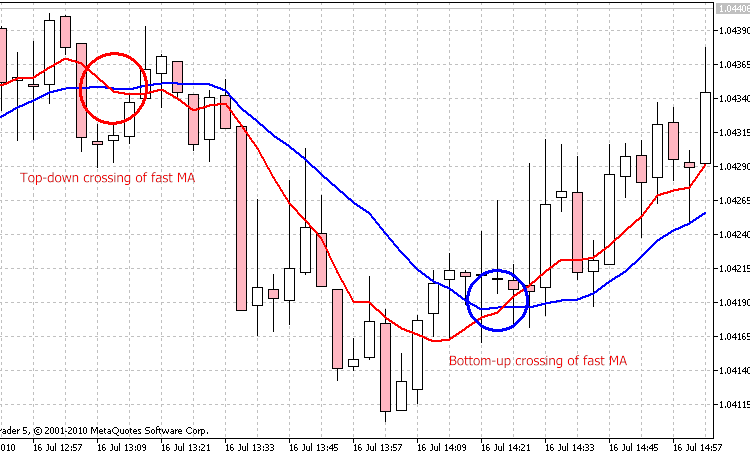Figure 1. The intersection of two moving averages

Using the TradeSignal_01() function, we'll get a signal about the intersection of two Moving Averages (МА): the fast one with period 8 and the slow one with period 16.

In our function we will fix only the fact of intersection and return the value of the corresponding signal to the function. According to our rules and prototype, the function will look like:

```int TradeSignal_01()
{
//--- zero means that there is no signal
int sig=0;

//--- check the handles of indicators
if(h_ma1==INVALID_HANDLE)//--- if the handle is invalid
{
//--- create is again
h_ma1=iMA(Symbol(),Period(),8,0,MODE_SMA,PRICE_CLOSE);
//--- exit from the function
return(0);
}
else //--- if the handle is valid
{
//--- copy value of the indicator to the array
if(CopyBuffer(h_ma1,0,0,3,ma1_buffer)<3) //--- if the array of data is less than required
//--- exit from the function
return(0);
//--- set the indexation in the array as in a timeseries
if(!ArraySetAsSeries(ma1_buffer,true))
//--- in case of an indexation error, exit from the function
return(0);
}

if(h_ma2==INVALID_HANDLE)//--- if the handle is invalid
{
//--- create it again
h_ma2=iMA(Symbol(),Period(),16,0,MODE_SMA,PRICE_CLOSE);
//--- exit from the function
return(0);
}
else //--- if the handle is valid
{
//--- copy values of the indicator to the array
if(CopyBuffer(h_ma2,0,0,2,ma2_buffer)<2) //--- if there is less data than required
//--- exit from the function
return(0);
//--- set the indexation in the array as in a timeseries
if(!ArraySetAsSeries(ma1_buffer,true))
//--- in case of an indexation error, exit from the function
return(0);
}

//--- check the condition and set a value for the sig
if(ma1_buffer<ma2_buffer && ma1_buffer>ma2_buffer)
sig=1;
else if(ma1_buffer>ma2_buffer && ma1_buffer<ma2_buffer)
sig=-1;
else sig=0;

return(sig);
}```

Now let's take a more detailed look at every part of the code. At the beginning of the function we declare a local variable that will store the type of signal, and initialize it with zero what means the absence of signal.

`int sig=0;`

Further, we check the validity of the handle; if the handle is invalid then we create it and exit from the function, because the calculation of the indicator takes some time. It is implemented to avoid the error of copying data from the indicator buffer.

```   if(h_ma1==INVALID_HANDLE)//--- if the handle is invalid
{
//--- create it again
h_ma1=iMA(Symbol(),Period(),8,0,MODE_SMA,PRICE_CLOSE);
//--- exit from the function
return(0);
}```

If the handle is valid, copy the data to the array. To analyze the situation, it's enough to copy the data of the last three bars of the fast MA an two bars of the slow one. Use the following function for this purpose:

```int  CopyBuffer(
int       indicator_handle,     // handle of an indicator
int       buffer_num,           // number of buffer of an indicator
int       start_pos,            // where to start from
int       count,                // amount to be copied
double    buffer[]              // an array to copy data to
);
```

Check them: if there is less data than required, it means that an error of copying has occurred; and further referring to the array where the data should have been stored will lead to an error. To avoid it, we exit from the function. Also we need to set the indexation in the array like in a timeseries; the following function is intended for it:

```bool  ArraySetAsSeries(
void  array[],     // array by a link
bool  set          // true means that the indexation order is reversed
);
```

If an error occurred during the indexation of the array, exit from the function, otherwise we can get incorrect results.

```else //--- if the handle is valid
{
//--- copy values of the indicator to the array
if(CopyBuffer(h_ma1,0,0,3,ma1_buffer)<3) //--- if there is less data than required
//--- exit from the function
return(0);
//--- set the indexation in the array like in a timeseries
if(!ArraySetAsSeries(ma1_buffer,true))
//--- in case of an indexation error, exit from the function
return(0);
}
```

Now, as the indicators are created and all the necessary information is obtained, let's proceed to the main stage - forming a signal.

To eliminate flickering of the signal at the current bar, analyze only the closed 1-st and 2-nd bars.

Form the signal to buy. For this purpose, take the value of the fast MA at the 2-nd bar and compare it to the value of the slow MA at the 1-bar; and compare the value of the fast MA at the 1-st bar with the value of the slow MA at the 1-st bar. If the value of the fast MA at the 2-nd bar is less than the value of the slow MA at the 1-st bar, and the value of the fast MA at the 1-st bar is greater than the value of the slow MA at the 1-st bar, it means that the fast MA crossed the slow one upwards; that is our signal to buy. If our condition is true, then write 1 to the sig variable.

The signal to sell is formed in the similar way. If the fast MA at the 2-nd bar is greater than the slow MA at the 1-st bar, and if the fast MA at 1-st bar is less than the slow MA at the 1-st bar, it means top-down crossing of the slow MA by the fast MA. If our condition is true, write value -1 to the sig variable. If both conditions are false, it means that there is no signal, so we write value 0 to the sig variable. Now the signals are formed, return the obtained signal type to our function TradeSignal_01()

```//--- check the condition and set a value for the sig
if(ma1_buffer<ma2_buffer && ma1_buffer>ma2_buffer)
sig=1;
else if(ma1_buffer>ma2_buffer && ma1_buffer<ma2_buffer)
sig=-1;
else sig=0;

return(sig);```

3.2. Intersection of the Main and Signal Line of MACD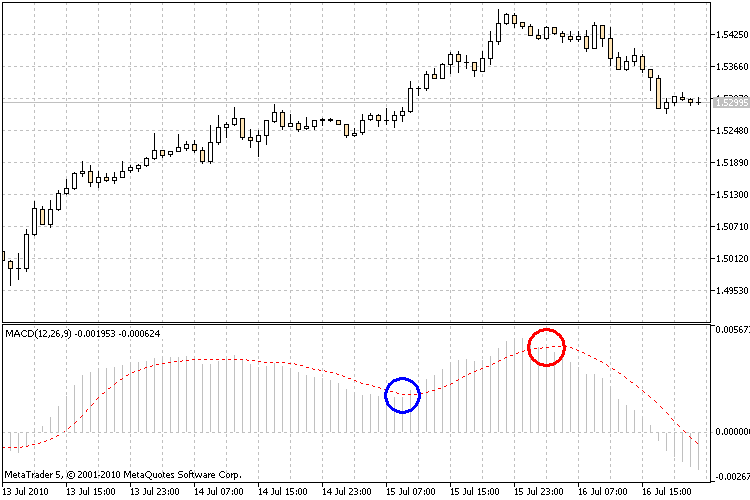Figure 2. The intersection of the main and signal line of the MACD indicator

In the TradeSignal_02() function we will get the signal about the intersection of the signal and the main line of MACD. If the signal line crosses the main one from top downwards, it is the signal  to buy. If the signal line crosses the main one bottom-up, it is the signal to sell. Other cases will be considered as the absence of signal.

```int TradeSignal_02()
{
int sig=0;

if(h_macd==INVALID_HANDLE)
{
h_macd=iMACD(Symbol(),Period(),12,26,9,PRICE_CLOSE);
return(0);
}
else
{
if(CopyBuffer(h_macd,0,0,2,macd1_buffer)<2)
return(0);
if(CopyBuffer(h_macd,1,0,3,macd2_buffer)<3)
return(0);
if(!ArraySetAsSeries(macd1_buffer,true))
return(0);
if(!ArraySetAsSeries(macd2_buffer,true))
return(0);
}

//--- check the condition and set a value for sig
if(macd2_buffer>macd1_buffer && macd2_buffer<macd1_buffer)
sig=1;
else if(macd2_buffer<macd1_buffer && macd2_buffer>macd1_buffer)
sig=-1;
else sig=0;

return(sig);
}```

3.3. Breakthrough of the Price Channel Range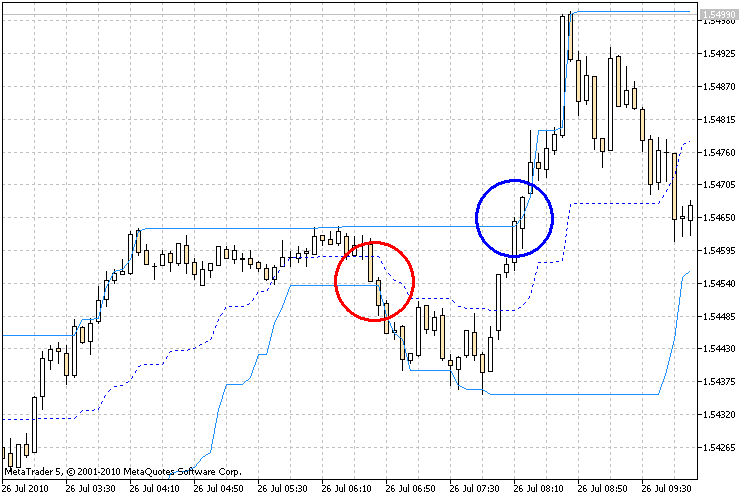Figure 3. Breakthrough of the lower and upper borders of the Price Channel

In the TradeSignal_03() function we will receive the signal about a breakthrough of the upper or lower borders of the Price Channel.

If the price pierces the upper border of the Price Channel and the price is fixed above this border, it is the signal to buy. If the price pierces the lower border of the Price Channel and the price is fixed below this border, then it's the signal to sell. Other cases are considered as the absence of signal.

Unlike in two previous functions, here we need an array for storing the close prices. Use the following function to get them:

```int  CopyClose(
string           symbol_name,       // symbol name
ENUM_TIMEFRAMES  timeframe,          // period
int              start_pos,         // where to start from
int              count,             // amount to be copied
double           close_array[]      // array for copying close prices to
);
```
```int TradeSignal_03()
{
int sig=0;

if(h_pc==INVALID_HANDLE)
{
h_pc=iCustom(Symbol(),Period(),"Price Channel",22);
return(0);
}
else
{
if(CopyBuffer(h_pc,0,0,3,pc1_buffer)<3)
return(0);
if(CopyBuffer(h_pc,1,0,3,pc2_buffer)<3)
return(0);
if(CopyClose(Symbol(),Period(),0,2,Close)<2)
return(0);
if(!ArraySetAsSeries(pc1_buffer,true))
return(0);
if(!ArraySetAsSeries(pc2_buffer,true))
return(0);
if(!ArraySetAsSeries(Close,true))
return(0);
}
//--- check the conditions and set a value for sig
if(Close>pc1_buffer)
sig=1;
else if(Close<pc2_buffer)
sig=-1;
else sig=0;

return(sig);
}```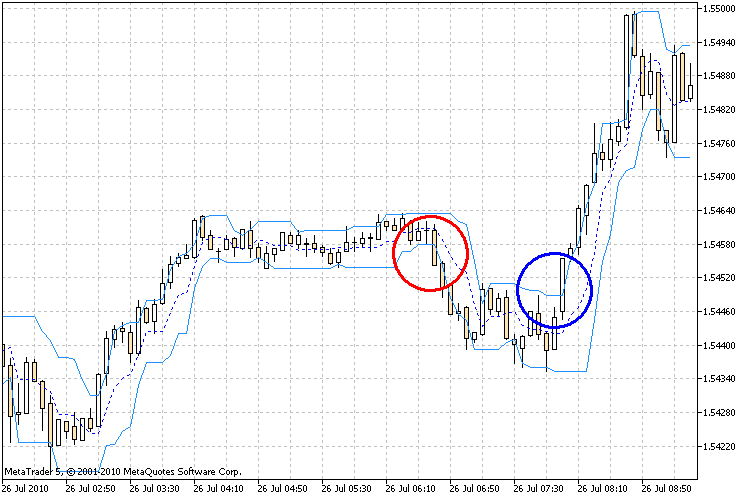Figure 4. The breakthrough of the upper and lower borders of the ADX adaptive channel.

Using the TradeSignal_04() function we will get the signal about a breakthrough of the upper or lower borders of the ADX adaptive channel.

If the price pierces the upper border of the adaptive channel ADX and the close price is fixed above this border, it is the signal to buy. If the price pierces the lower border of the Price Channel and the close price is fixed below this border, it is the signal to sell. Other cases are considered as the absence of signal.

```int TradeSignal_04()
{
int sig=0;

{
return(0);
}
else
{
return(0);
return(0);
if(CopyClose(Symbol(),Period(),0,2,Close)<2)
return(0);
return(0);
return(0);
if(!ArraySetAsSeries(Close,true))
return(0);
}
//--- check the condition and set a value for the sig
sig=1;
sig=-1;
else sig=0;

return(sig);
}```

3.5. Exit from the Overbuying/Overselling Zones of Stochastic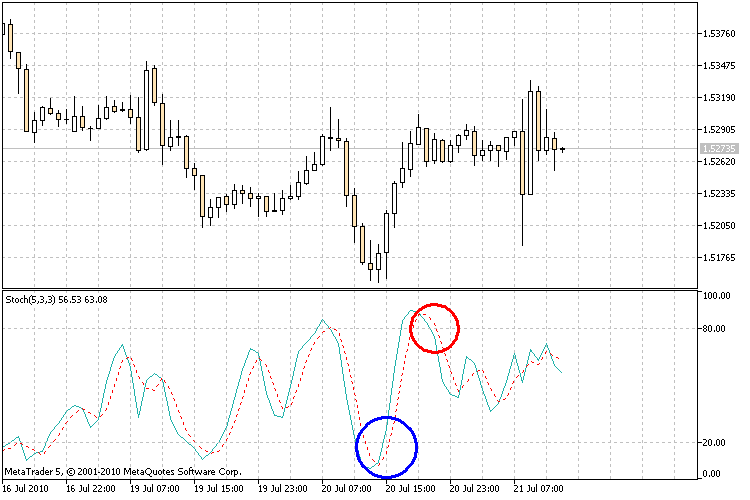Figure 5. Crossing of the overbuying and overselling levels by the stochastic.

Using the TradeSignal_05() we will get the signal about exiting of the stochastic from the zones of overbuying/overselling; levels of those zones will be levels with values 80 and 20.

We buy, as the oscillator (%K or %D) falls below a certain level (usually, it is 20) and then rises above it. We sell, as the oscillator rises higher than a certain level (usually, it is 80) and then fall below it.

```int TradeSignal_05()
{
int sig=0;

if(h_stoh==INVALID_HANDLE)
{
h_stoh=iStochastic(Symbol(),Period(),5,3,3,MODE_SMA,STO_LOWHIGH);
return(0);
}
else
{
if(CopyBuffer(h_stoh,0,0,3,stoh_buffer)<3)
return(0);

if(!ArraySetAsSeries(stoh_buffer,true))
return(0);
}
//--- check the condition and set a value for sig
if(stoh_buffer<20 && stoh_buffer>20)
sig=1;
else if(stoh_buffer>80 && stoh_buffer<80)
sig=-1;
else sig=0;

return(sig);
}```

3.6. Exit from the Overbuying/Overselling Zones of RSI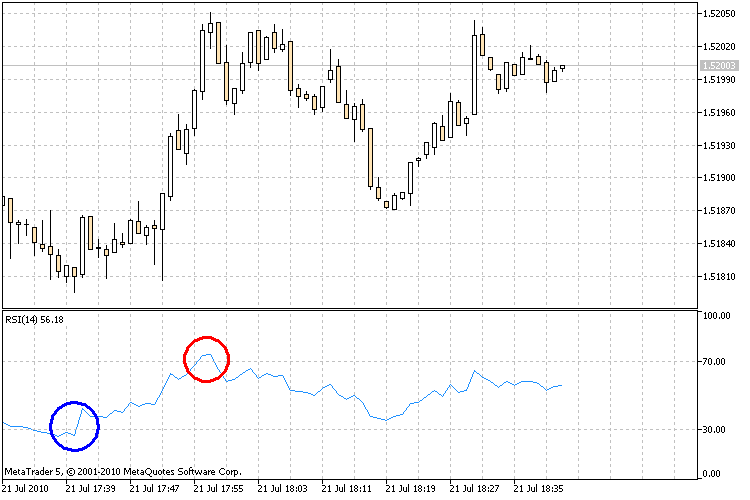Figure 6. Crossing of the overbuying and overselling levels by the RSI indicator

Using the TradeSignal_06() function we will get the signal about exiting of the RSI indicator from the zones of overbuying/overselling; levels for that zones are the levels with values 70 and 30.

We buy, as RSI fall lower than a certain level (usually, it is 30) and then rises above it. We sell, as RSI rises higher than a certain level (usually, it is 70) and then falls below it.

```int TradeSignal_06()
{
int sig=0;

if(h_rsi==INVALID_HANDLE)
{
h_rsi=iRSI(Symbol(),Period(),14,PRICE_CLOSE);
return(0);
}
else
{
if(CopyBuffer(h_rsi,0,0,3,rsi_buffer)<3)
return(0);

if(!ArraySetAsSeries(rsi_buffer,true))
return(0);
}
//--- check the condition and set a value for sig
if(rsi_buffer<30 && rsi_buffer>30)
sig=1;
else if(rsi_buffer>70 && rsi_buffer<70)
sig=-1;
else sig=0;

return(sig);

```
3.7. Exit from the Overbuying/Overselling Zones of CCI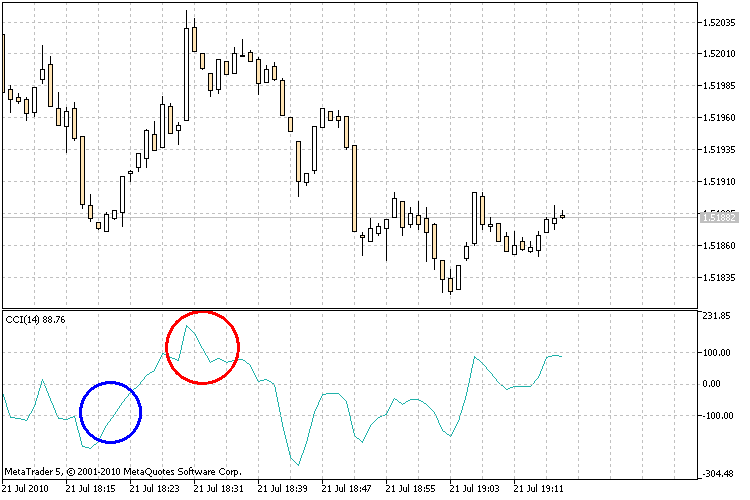Figure 7. Crossing of the overbuying and overselling levels by the CCI indicator

Using the TradeSignal_07() we will get the signal about exiting of the CCI indicator from the zones of overbuying/overselling; levels for that zones are the levels with values 100 and -100.

We buy, as CCI fall lower then level -100 and then rises above it. We sell, as CCI rises above level 100 and then falls below it.

```int TradeSignal_07()
{
int sig=0;

if(h_cci==INVALID_HANDLE)
{
h_cci=iCCI(Symbol(),Period(),14,PRICE_TYPICAL);
return(0);
}
else
{
if(CopyBuffer(h_cci,0,0,3,cci_buffer)<3)
return(0);

if(!ArraySetAsSeries(cci_buffer,true))
return(0);
}
//--- check the condition and set a value for sig
if(cci_buffer<-100 && cci_buffer>-100)
sig=1;
else if(cci_buffer>100 && cci_buffer<100)
sig=-1;
else sig=0;

return(sig);
}```

3.8. Exit from the Overbuying/Overselling Zones of Williams %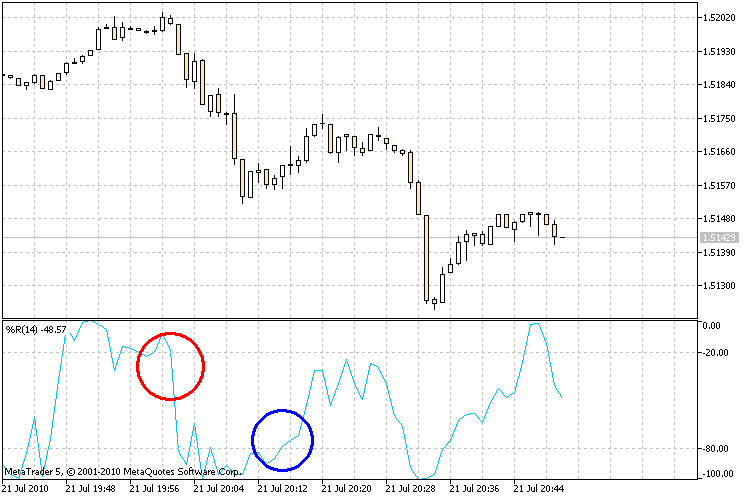Figure 8. Crossing of the overbuying and overselling levels by the Williams % indicator

Using the TradeSignal_08() function we will get the signal about exiting of the Williams % indicator from the zones of overbuying/overselling; levels for that zones are the levels with values -20 and -80.

We buy, as Williams % falls lower than level -80 and then rises above it. We sell, as Williams % rises above level -20 and then falls below it.

```int TradeSignal_08()
{
int sig=0;

if(h_wpr==INVALID_HANDLE)
{
h_wpr=iWPR(Symbol(),Period(),14);
return(0);
}
else
{
if(CopyBuffer(h_wpr,0,0,3,wpr_buffer)<3)
return(0);

if(!ArraySetAsSeries(wpr_buffer,true))
return(0);
}
//--- check the condition and set a value for sig
if(wpr_buffer<-80 && wpr_buffer>-80)
sig=1;
else if(wpr_buffer>-20 && wpr_buffer<-20)
sig=-1;
else sig=0;

return(sig);
}```
3.9. Bounce from the Borders of the Bollinger Channel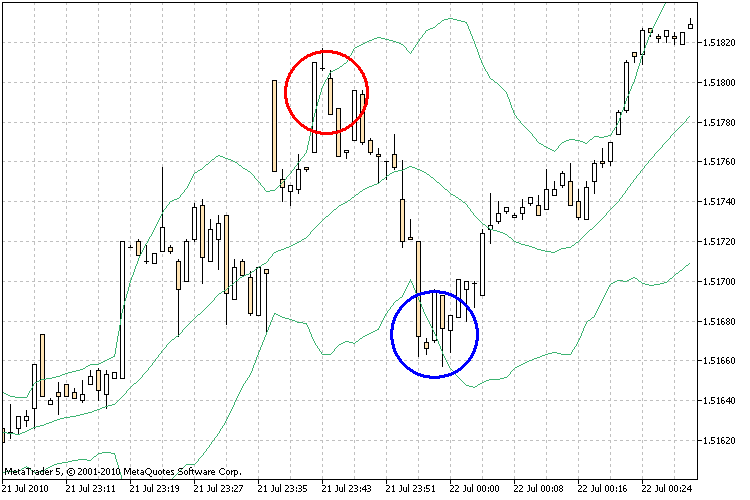Figure 9. Bounce of the price from the border of the Bollinger channel

Using the TradeSignal_09() function we will get the signal when the price bounces from the borders of the Bollinger channel.

If the price pierces or touches the upper border of the Bollinger and then returns back, it is the signal to sell. If the price pierces or touches the lower border of the Bollinger, then it's the signal to buy.

```int TradeSignal_09()
{
int sig=0;

if(h_bb==INVALID_HANDLE)
{
h_bb=iBands(Symbol(),Period(),20,0,2,PRICE_CLOSE);
return(0);
}
else
{
if(CopyBuffer(h_bb,1,0,2,bb1_buffer)<2)
return(0);
if(CopyBuffer(h_bb,2,0,2,bb2_buffer)<2)
return(0);
if(CopyClose(Symbol(),Period(),0,3,Close)<3)
return(0);
if(!ArraySetAsSeries(bb1_buffer,true))
return(0);
if(!ArraySetAsSeries(bb2_buffer,true))
return(0);
if(!ArraySetAsSeries(Close,true))
return(0);
}
//--- check the condition and set a value for sig
if(Close<=bb2_buffer && Close>bb2_buffer)
sig=1;
else if(Close>=bb1_buffer && Close<bb1_buffer)
sig=-1;
else sig=0;

return(sig);
}```

3.10. Bounce from the Borders of the Standard Deviation Channel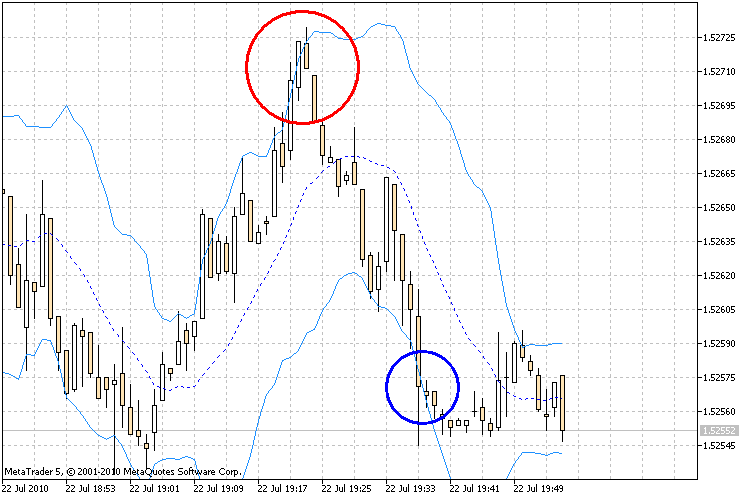Figure 10. Bounce of the price from the borders of the standard deviation channel

Using the TradeSignal_10() function we will get the signal if the price bounce from the borders of the standard deviation channel.

If the price pierces or touches the upper border of the standard deviation channel and then returns back, it's the signal to sell. If the price pierces or touches the lower border of the standard deviation channel, then it's the signal to buy.

```int TradeSignal_10()
{
int sig=0;

if(h_sdc==INVALID_HANDLE)
{
h_sdc=iCustom(Symbol(),Period(),"StandardDeviationChannel",14,0,MODE_SMA,PRICE_CLOSE,2.0);
return(0);
}
else
{
if(CopyBuffer(h_sdc,0,0,2,sdc1_buffer)<2)
return(0);
if(CopyBuffer(h_sdc,1,0,2,sdc2_buffer)<2)
return(0);
if(CopyClose(Symbol(),Period(),0,3,Close)<3)
return(0);
if(!ArraySetAsSeries(sdc1_buffer,true))
return(0);
if(!ArraySetAsSeries(sdc2_buffer,true))
return(0);
if(!ArraySetAsSeries(Close,true))
return(0);
}
//--- check the condition and set a value for sig
if(Close<=sdc2_buffer && Close>sdc2_buffer)
sig=1;
else if(Close>=sdc1_buffer && Close<sdc1_buffer)
sig=-1;
else sig=0;

return(sig);
}```

3.11. Bounce from the Borders of the Price Channel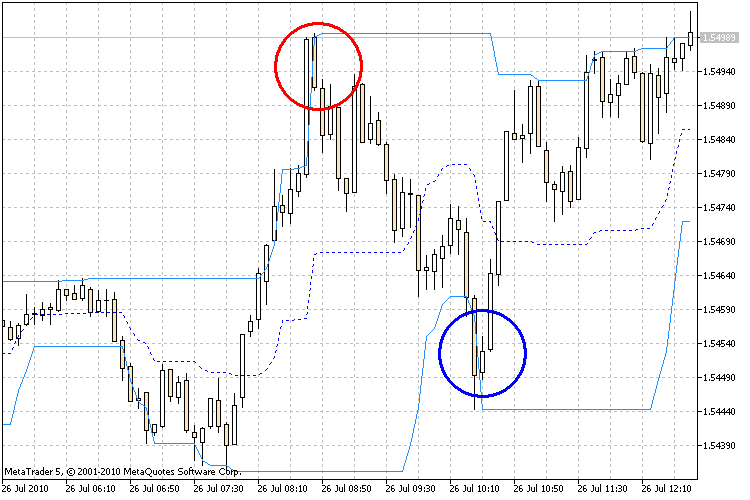Figure 11. The bounce of price from the borders of the Price Channel

Using the TradeSignal_11() function we will get the signal if the price bounces from the borders of the Price Channel.

If the price pierces or touches the upper border of the Price Channel and then returns back, it's the signal for selling. If the price pierces or touches the lower border of the Price Channel, then it's the signal to buy.

```int TradeSignal_11()
{
int sig=0;

if(h_pc==INVALID_HANDLE)
{
h_pc=iCustom(Symbol(),Period(),"Price Channel",22);
return(0);
}
else
{
if(CopyBuffer(h_pc,0,0,4,pc1_buffer)<4)
return(0);
if(CopyBuffer(h_pc,1,0,4,pc2_buffer)<4)
return(0);
if(CopyClose(Symbol(),Period(),0,3,Close)<3)
return(0);
if(!ArraySetAsSeries(pc1_buffer,true))
return(0);
if(!ArraySetAsSeries(pc2_buffer,true))
return(0);
if(!ArraySetAsSeries(Close,true))
return(0);
}
//--- check the condition and set a value for sig
if(Close>pc2_buffer && Close<=pc2_buffer)
sig=1;
else if(Close<pc1_buffer && Close>=pc1_buffer)
sig=-1;
else sig=0;

return(sig);
}```

3.12. Bounce from the Borders of the Envelopes Channel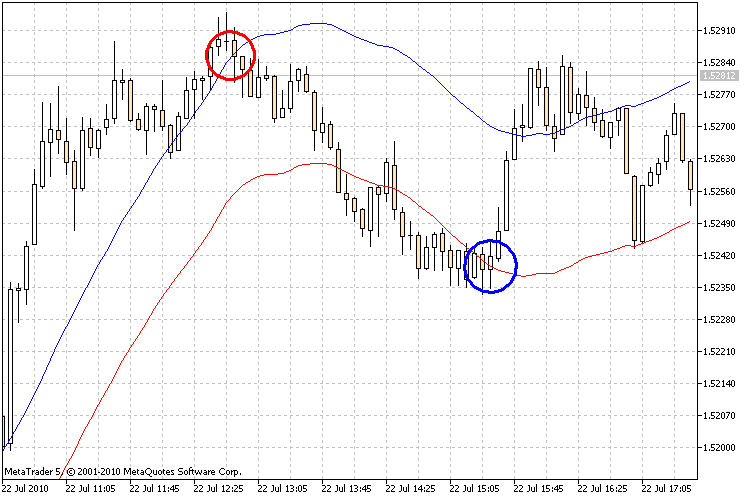Figure 12. The bounce of price from the borders of the Envelopes channel

Using the TradeSignal_12() function we will get the signal if the price bounces from the borders of the Envelopes channel.

If the price pierces or touches the upper border of the Envelopes channel and then returns back, it's the signal to sell. If the price pierces or touches the lower border of the Envelopes channel, it's the signal to buy.

```int TradeSignal_12()
{
int sig=0;

if(h_env==INVALID_HANDLE)
{
h_env=iEnvelopes(Symbol(),Period(),28,0,MODE_SMA,PRICE_CLOSE,0.1);
return(0);
}
else
{
if(CopyBuffer(h_env,0,0,2,env1_buffer)<2)
return(0);
if(CopyBuffer(h_env,1,0,2,env2_buffer)<2)
return(0);
if(CopyClose(Symbol(),Period(),0,3,Close)<3)
return(0);
if(!ArraySetAsSeries(env1_buffer,true))
return(0);
if(!ArraySetAsSeries(env2_buffer,true))
return(0);
if(!ArraySetAsSeries(Close,true))
return(0);
}
//--- check the condition and set a value for sig
if(Close<=env2_buffer && Close>env2_buffer)
sig=1;
else if(Close>=env1_buffer && Close<env1_buffer)
sig=-1;
else sig=0;

return(sig);
}```

3.13. Breakthrough of the Donchian Channel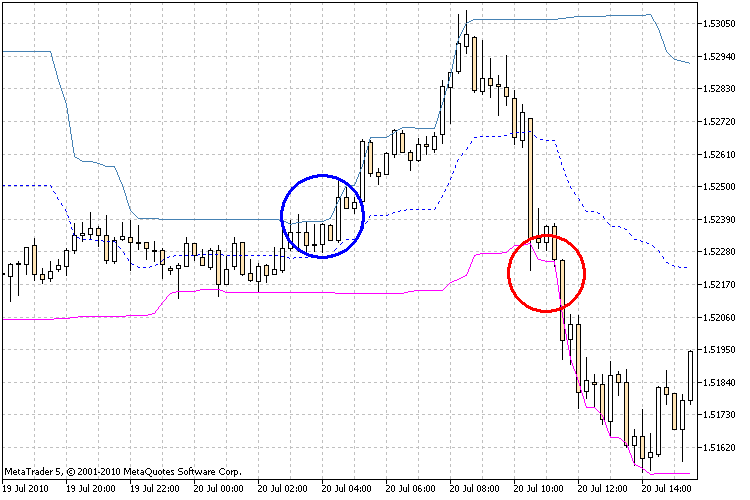Figure 13. Breakthrough of the borders of the Donchian Channel

In the TradeSignal_13() function we will get the signal when the price pierces the borders of the Donchian channel.

If the price pierces the upper border of the Donchian channel and the close price is fixed above that border, it is the signal to buy. If the price pierces the lower border of the Donchian channel and the close price is fixed below this border, it is the signal to sell.

```int TradeSignal_13()
{
int sig=0;

if(h_dc==INVALID_HANDLE)
{
h_dc=iCustom(Symbol(),Period(),"Donchian Channels",24,3,-2);
return(0);
}
else
{
if(CopyBuffer(h_dc,0,0,3,dc1_buffer)<3)
return(0);
if(CopyBuffer(h_dc,1,0,3,dc2_buffer)<3)
return(0);
if(CopyClose(Symbol(),Period(),0,2,Close)<2)
return(0);
if(!ArraySetAsSeries(dc1_buffer,true))
return(0);
if(!ArraySetAsSeries(dc2_buffer,true))
return(0);
if(!ArraySetAsSeries(Close,true))
return(0);
}
//--- check the condition and set a value for sig
if(Close>dc1_buffer)
sig=1;
else if(Close<dc2_buffer)
sig=-1;
else sig=0;

return(sig);
}```

3.14. Breakthrough of the Silver-Channel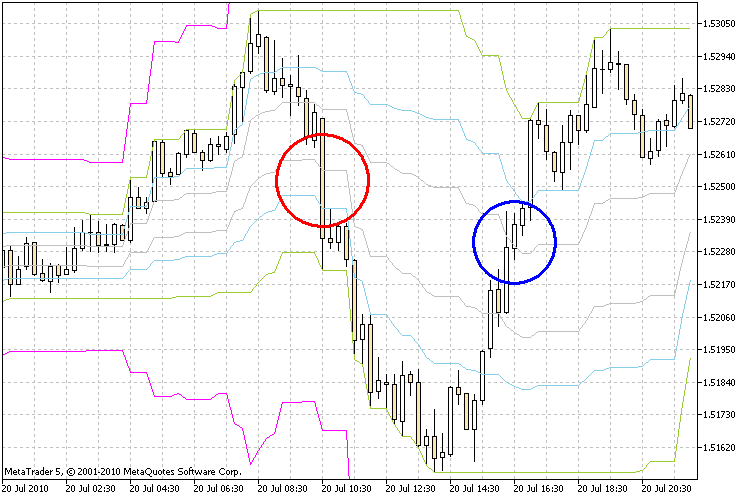Figure 14. Breakthrough of the borders of the Silver-Channel

In the TradeSignal_14() function we will get the signal when the price pierces the borders of the Silver-Channel.  The Silver-Channel indicator draws 8 borders that can also serve as support and resistance levels. To obtain the signal we will use 2 middle borders.

If the price pierces the upper border of the Silver-Channel and the close price is fixed above this border, it's the signal to buy. If the price pierces the lower border of the Silver-Channel and the close price is fixed below this border, it is the signal to sell.

```int TradeSignal_14()
{
int sig=0;

if(h_sc==INVALID_HANDLE)
{
h_sc=iCustom(Symbol(),Period(),"Silver-channels",26,38.2,23.6,0,61.8);
return(0);
}
else
{
if(CopyBuffer(h_sc,0,0,2,sc1_buffer)<2)
return(0);
if(CopyBuffer(h_sc,1,0,2,sc2_buffer)<2)
return(0);
if(CopyClose(Symbol(),Period(),0,3,Close)<3)
return(0);
if(!ArraySetAsSeries(sc1_buffer,true))
return(0);
if(!ArraySetAsSeries(sc2_buffer,true))
return(0);
if(!ArraySetAsSeries(Close,true))
return(0);
}
//--- check the condition and set a value for sig
if(Close<sc1_buffer && Close>sc1_buffer)
sig=1;
else if(Close>sc2_buffer && Close<sc2_buffer)
sig=-1;
else sig=0;

return(sig);
}```

3.15. Breakthrough of the Gallagher Channel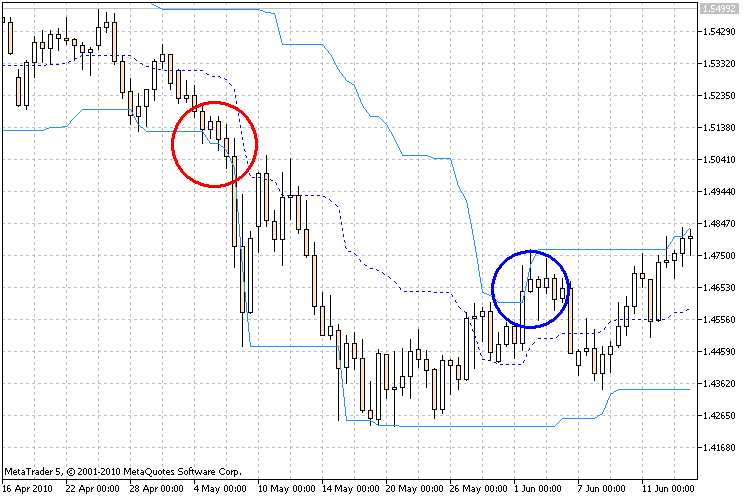Figure 15. Breakthrough of the borders of the Gallagher channel

In the TradeSignal_15() channel we will get the signal when the price pierces the borders of the Gallagher channel. The indicator of the Gallagher channel is drawn by maximums and minimums for 10 days.

If the price pierces the upper border of the Gallagher channel and the close price is fixed above this border, it is the signal to buy. If the price pierces the lower border of the Gallagher channel and the close price is fixed below this border, it is the signal to sell.

```int TradeSignal_15()
{
int sig=0;

if(h_gc==INVALID_HANDLE)
{
h_gc=iCustom(Symbol(),Period(),"PriceChannelGalaher");
return(0);
}
else
{
if(CopyBuffer(h_gc,0,0,3,gc1_buffer)<3)
return(0);
if(CopyBuffer(h_gc,1,0,3,gc2_buffer)<3)
return(0);
if(CopyClose(Symbol(),Period(),0,2,Close)<2)
return(0);
if(!ArraySetAsSeries(gc1_buffer,true))
return(0);
if(!ArraySetAsSeries(gc2_buffer,true))
return(0);
if(!ArraySetAsSeries(Close,true))
return(0);
}
//--- check the condition and set a value for sig
if(Close>gc1_buffer)
sig=1;
else if(Close<gc2_buffer)
sig=-1;
else sig=0;

return(sig);
}```

3.16. Change of Trend by NRTR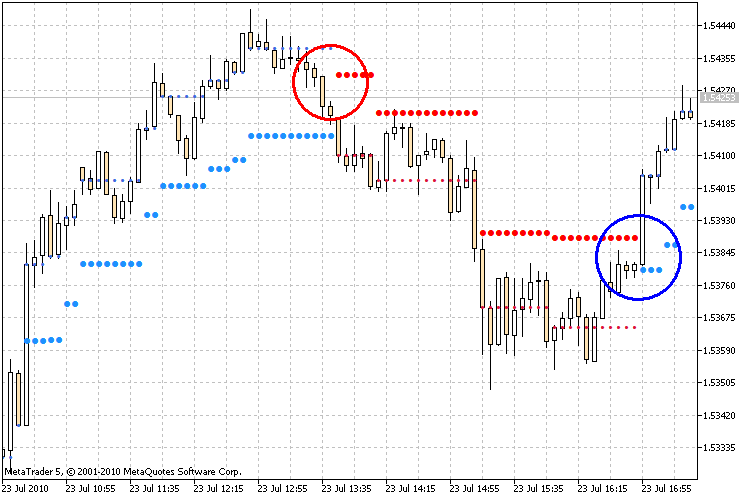Figure 16. Identification of a trend change using the NRTR indicator

In the TradeSignal_16() function we will get the signal when the NRTR trend changes.

If the NRTR indicator shows a rising trend, it's the signal to buy. If NRTR shows a descending trend, it's the signal to sell.

```int TradeSignal_16()
{
int sig=0;

if(h_nrtr==INVALID_HANDLE)
{
h_nrtr=iCustom(Symbol(),Period(),"NRTR",40,2.0);
return(0);
}
else
{
if(CopyBuffer(h_nrtr,0,0,2,nrtr1_buffer)<2)
return(0);
if(CopyBuffer(h_nrtr,1,0,2,nrtr2_buffer)<2)
return(0);
if(!ArraySetAsSeries(nrtr1_buffer,true))
return(0);
if(!ArraySetAsSeries(nrtr2_buffer,true))
return(0);
}
//--- check the condition and set a value for sig
if(nrtr1_buffer>0)
sig=1;
else if(nrtr2_buffer>0)
sig=-1;
else sig=0;

return(sig);
}```

3.17. Change of Trend by Alligator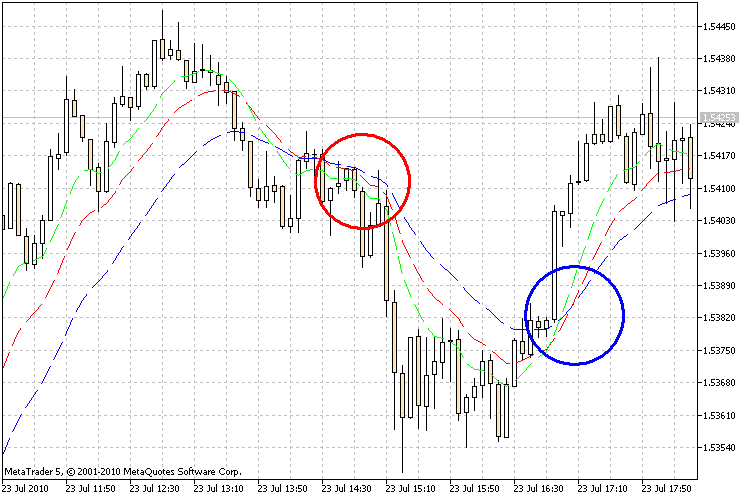Figure 17. Change of the trend by Alligator

In the TradeSignal_17() function we will get the signal when the Alligator trend changes.

If the Jaw, Teeth and Lips are closed and kinked, the Alligator is going to sleep or is already sleeping. When it sleeps, its hunger grows; and the more it sleeps, the hungrier it is when wakes up. The first thing it does when wakes up is it opens its mouth and yawns. Then it starts smelling the food - meat of a bull or a bear, and then it starts hunting it. As soon as the Alligator has eaten enough, it loses its interest to food-price (Balance Lines converge); it is the time to fix the profit.

```int TradeSignal_17()
{
int sig=0;

if(h_al==INVALID_HANDLE)
{
h_al=iAlligator(Symbol(),Period(),13,0,8,0,5,0,MODE_SMMA,PRICE_MEDIAN);
return(0);
}
else
{
if(CopyBuffer(h_al,0,0,2,al1_buffer)<2)
return(0);
if(CopyBuffer(h_al,1,0,2,al2_buffer)<2)
return(0);
if(CopyBuffer(h_al,2,0,2,al3_buffer)<2)
return(0);
if(!ArraySetAsSeries(al1_buffer,true))
return(0);
if(!ArraySetAsSeries(al2_buffer,true))
return(0);
if(!ArraySetAsSeries(al3_buffer,true))
return(0);
}
//--- check the condition and set a value for sig
if(al3_buffer>al2_buffer && al2_buffer>al1_buffer)
sig=1;
else if(al3_buffer<al2_buffer && al2_buffer<al1_buffer)
sig=-1;
else sig=0;

return(sig);
}```

3.18. Change of Trend by AMA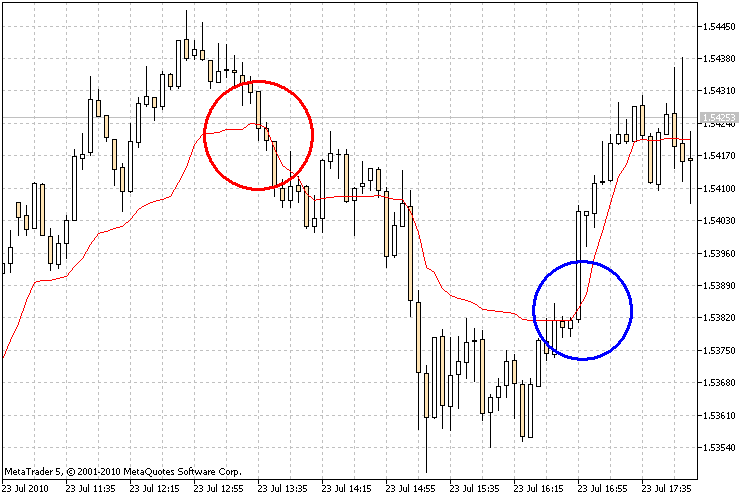Figure 18. Change of the trend by AMA

In the TradeSignal_18() function we will get the signal when the AMA trend changes.

If the AMA indicator is directed upwards, it's the signal to buy. If the AMA is directed downwards, then it's the signal to sell.

```int TradeSignal_18()
{
int sig=0;

if(h_ama==INVALID_HANDLE)
{
h_ama=iAMA(Symbol(),Period(),9,2,30,0,PRICE_CLOSE);
return(0);
}
else
{
if(CopyBuffer(h_ama,0,0,3,ama_buffer)<3)
return(0);
if(!ArraySetAsSeries(ama_buffer,true))
return(0);
}
//--- check the condition and set a value for sig
if(ama_buffer<ama_buffer)
sig=1;
else if(ama_buffer>ama_buffer)
sig=-1;
else sig=0;

return(sig);
}```

3.19. Change of Color of the Awesome Oscillator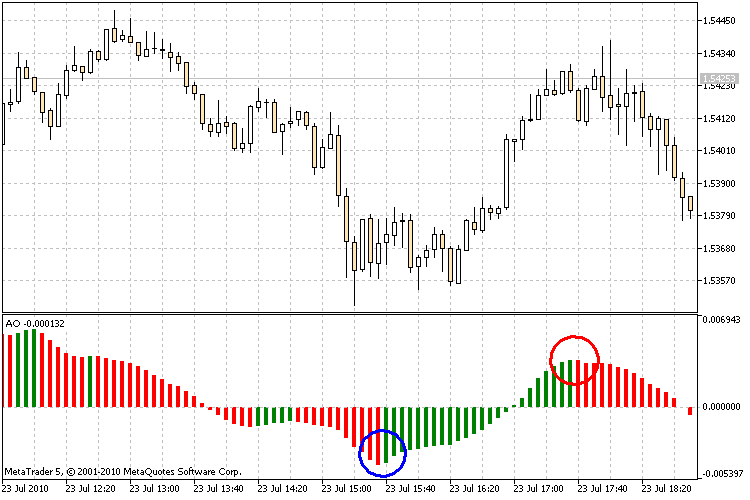Figure 19. Identification of a trend change using the Awesome Oscillator indicator

In the TradeSignal_19() function we will get the signal when the color of the Awesome Oscillator histogram changes.

One of features of MQL5 is the possibility of creating buffers for indicators, where one can store indexes of the color of lines set in the #property indicator_colorN properties. When the color of the Awesome Oscillator histogram is green, it's the signal to buy. If its color is changed to red, it's the signal to sell.

```int TradeSignal_19()
{
int sig=0;

if(h_ao==INVALID_HANDLE)
{
h_ao=iAO(Symbol(),Period());
return(0);
}
else
{
if(CopyBuffer(h_ao,1,0,20,ao_buffer)<20)
return(0);
if(!ArraySetAsSeries(ao_buffer,true))
return(0);
}
//--- check the condition and set a value for sig
if(ao_buffer==0)
sig=1;
else if(ao_buffer==1)
sig=-1;
else sig=0;

return(sig);
}```

3.20. Change of Trend by Ichimoku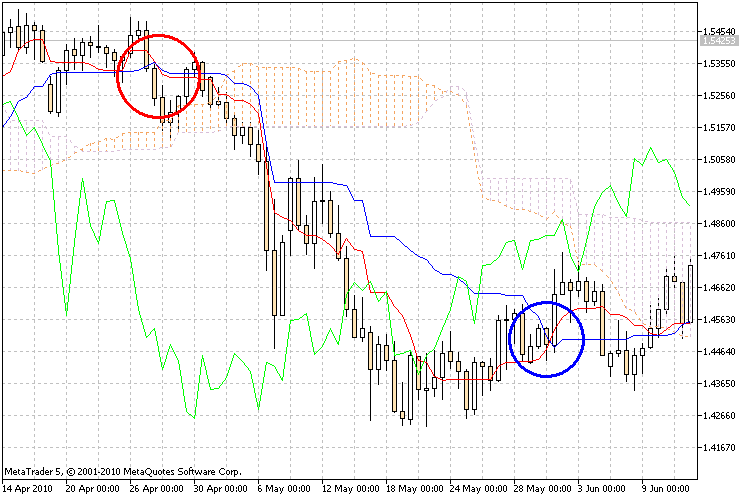Figure 20. Identification of a trend change using the Ichimoku indicator

In the TradeSignal_20() function we will get the signal when the Ichimoku trend changes. For this purpose we will analyze intersection of the Tenkan-sen and Kijun-sen lines.

The signal to buy is generated when the Tenkan-sen line crosses the Kijun-sen bottom-up. The top-down crossing is the signal to sell.

```int TradeSignal_20()
{
int sig=0;

if(h_ich==INVALID_HANDLE)
{
h_ich=iIchimoku(Symbol(),Period(),9,26,52);
return(0);
}
else
{
if(CopyBuffer(h_ich,0,0,2,ich1_buffer)<2)
return(0);
if(CopyBuffer(h_ich,1,0,2,ich2_buffer)<2)
return(0);
if(!ArraySetAsSeries(ich1_buffer,true))
return(0);
if(!ArraySetAsSeries(ich2_buffer,true))
return(0);
}
//--- check the condition and set a value for sig
if(ich1_buffer>ich2_buffer)
sig=1;
else if(ich1_buffer<ich2_buffer)
sig=-1;
else sig=0;

return(sig);
}```

### 4. Make It as an Indicator

Now, as we have the ready-made blocks of indicators, we can start writing an indicator that shows the signals on the basis of all the selected methods. Using the created template we can implement receiving of signal from any indicator; it's enough to correctly formulate a signal condition and add it to the code.

Let's write an indicator with inflexibly in-built parameters. Signals of the indicators will be drawn in the form of arrows (arrow up - but, down - sell, a cross - no signal) on the right part of the chart. Let's take the standard Wingdings font for drawing the arrows. Also we need to create several other functions for displaying the information about signals on the chart of a symbol. We will combine them in a separate block as a library that can be used for writing your own programs by adding new functions to it. Let's name this library LibFunctions.

In the header of our future indicator, write the connection of the file with functions for generating signal, and import function that are necessary for graphical displaying of signal, as well as declare variables on the global scape that will store the type of signal received from the indicator.

```//--- Connect necessary libraries of functions
//--- Import of functions from the LibFunctions library
#import "LibFunctions.ex5"
void SetLabel(string nm,string tx,ENUM_BASE_CORNER cn,ENUM_ANCHOR_POINT cr,int xd,int yd,string fn,int fs,double yg,color ct);
string arrow(int sig);
color Colorarrow(int sig);
#import
//+------------------------------------------------------------------+
//| Declare variables for storing signals of indicators              |
//+------------------------------------------------------------------+
int SignalMA;
int SignalMACD;
int SignalPC;
int SignalST;
int SignalRSI;
int SignalCCI;
int SignalWPR;
int SignalBB;
int SignalSDC;
int SignalPC2;
int SignalENV;
int SignalDC;
int SignalSC;
int SignalGC;
int SignalNRTR;
int SignalAL;
int SignalAMA;
int SignalAO;
int SignalICH;```

As I previously mentioned, the indicators are loaded to the terminal only once and the pointers (handles) to that indicators are created; that's why let's implement their creating in the OnInit() function, since this function is run only once at the program start.

```int OnInit()
{
//--- create indicator handles
h_ma1=iMA(Symbol(),Period(),8,0,MODE_SMA,PRICE_CLOSE);
h_ma2=iMA(Symbol(),Period(),16,0,MODE_SMA,PRICE_CLOSE);
h_macd=iMACD(Symbol(),Period(),12,26,9,PRICE_CLOSE);
h_pc=iCustom(Symbol(),Period(),"Price Channel",22);
h_stoh=iStochastic(Symbol(),Period(),5,3,3,MODE_SMA,STO_LOWHIGH);
h_rsi=iRSI(Symbol(),Period(),14,PRICE_CLOSE);
h_cci=iCCI(Symbol(),Period(),14,PRICE_TYPICAL);
h_wpr=iWPR(Symbol(),Period(),14);
h_bb=iBands(Symbol(),Period(),20,0,2,PRICE_CLOSE);
h_sdc=iCustom(Symbol(),Period(),"StandardDeviationChannel",14,0,MODE_SMA,PRICE_CLOSE,2.0);
h_env=iEnvelopes(Symbol(),Period(),28,0,MODE_SMA,PRICE_CLOSE,0.1);
h_dc=iCustom(Symbol(),Period(),"Donchian Channels",24,3,-2);
h_sc=iCustom(Symbol(),Period(),"Silver-channels",26,38.2,23.6,0,61.8);
h_gc=iCustom(Symbol(),Period(),"PriceChannelGalaher");
h_nrtr=iCustom(Symbol(),Period(),"NRTR",40,2.0);
h_al=iAlligator(Symbol(),Period(),13,0,8,0,5,0,MODE_SMMA,PRICE_MEDIAN);
h_ama=iAMA(Symbol(),Period(),9,2,30,0,PRICE_CLOSE);
h_ao=iAO(Symbol(),Period());
h_ich=iIchimoku(Symbol(),Period(),9,26,52);
return(0);
}```

All the main calculations are performed in the OnCalculate() function; there we will place the rest of indicator's code.

```int OnCalculate(const int rates_total,
const int prev_calculated,
const datetime &time[],
const double &open[],
const double &high[],
const double &low[],
const double &close[],
const long &tick_volume[],
const long &volume[],
{
//---assign the signal value to the variable

//--- draw graphical objects on the chart in the upper left corner
int size=((int)ChartGetInteger(0,CHART_HEIGHT_IN_PIXELS)/22);
int i=0;
int x=10;
int y=0;
int fz=size-4;

y+=size;
SetLabel("arrow"+(string)i,arrow(SignalMA),CORNER_RIGHT_UPPER,ANCHOR_RIGHT_UPPER,x,y+4,"Wingdings",fz-2,0,Colorarrow(SignalMA));
x+=size;
SetLabel("label"+(string)i,"Moving Average",CORNER_RIGHT_UPPER,ANCHOR_RIGHT_UPPER,x,y,"Arial",fz,0,BlueViolet);
i++;y+=size;x=10;
SetLabel("arrow"+(string)i,arrow(SignalMACD),CORNER_RIGHT_UPPER,ANCHOR_RIGHT_UPPER,x,y+4,"Wingdings",fz-2,0,Colorarrow(SignalMACD));
x+=size;
SetLabel("label"+(string)i,"MACD",CORNER_RIGHT_UPPER,ANCHOR_RIGHT_UPPER,x,y,"Arial",fz,0,BlueViolet);
i++;y+=size;x=10;
SetLabel("arrow"+(string)i,arrow(SignalPC),CORNER_RIGHT_UPPER,ANCHOR_RIGHT_UPPER,x,y+4,"Wingdings",fz-2,0,Colorarrow(SignalPC));
x+=size;
SetLabel("label"+(string)i,"Price Channell",CORNER_RIGHT_UPPER,ANCHOR_RIGHT_UPPER,x,y,"Arial",fz,0,BlueViolet);
i++;y+=size;x=10;
x+=size;
i++;y+=size;x=10;
SetLabel("arrow"+(string)i,arrow(SignalST),CORNER_RIGHT_UPPER,ANCHOR_RIGHT_UPPER,x,y+4,"Wingdings",fz-2,0,Colorarrow(SignalST));
x+=size;
SetLabel("label"+(string)i,"Stochastic Oscillator",CORNER_RIGHT_UPPER,ANCHOR_RIGHT_UPPER,x,y,"Arial",fz,0,BlueViolet);
i++;y+=size;x=10;
SetLabel("arrow"+(string)i,arrow(SignalRSI),CORNER_RIGHT_UPPER,ANCHOR_RIGHT_UPPER,x,y+4,"Wingdings",fz-2,0,Colorarrow(SignalRSI));
x+=size;
SetLabel("label"+(string)i,"RSI",CORNER_RIGHT_UPPER,ANCHOR_RIGHT_UPPER,x,y,"Arial",fz,0,BlueViolet);
i++;y+=size;x=10;
SetLabel("arrow"+(string)i,arrow(SignalCCI),CORNER_RIGHT_UPPER,ANCHOR_RIGHT_UPPER,x,y+4,"Wingdings",fz-2,0,Colorarrow(SignalCCI));
x+=size;
SetLabel("label"+(string)i,"CCI",CORNER_RIGHT_UPPER,ANCHOR_RIGHT_UPPER,x,y,"Arial",fz,0,BlueViolet);
i++;y+=size;x=10;
SetLabel("arrow"+(string)i,arrow(SignalWPR),CORNER_RIGHT_UPPER,ANCHOR_RIGHT_UPPER,x,y+4,"Wingdings",fz-2,0,Colorarrow(SignalWPR));
x+=size;
SetLabel("label"+(string)i,"WPR",CORNER_RIGHT_UPPER,ANCHOR_RIGHT_UPPER,x,y,"Arial",fz,0,BlueViolet);
i++;y+=size;x=10;
SetLabel("arrow"+(string)i,arrow(SignalBB),CORNER_RIGHT_UPPER,ANCHOR_RIGHT_UPPER,x,y+4,"Wingdings",fz-2,0,Colorarrow(SignalBB));
x+=size;
SetLabel("label"+(string)i,"Bollinger Bands",CORNER_RIGHT_UPPER,ANCHOR_RIGHT_UPPER,x,y,"Arial",fz,0,BlueViolet);
i++;y+=size;x=10;
SetLabel("arrow"+(string)i,arrow(SignalSDC),CORNER_RIGHT_UPPER,ANCHOR_RIGHT_UPPER,x,y+4,"Wingdings",fz-2,0,Colorarrow(SignalSDC));
x+=size;
SetLabel("label"+(string)i,"StDevChannel",CORNER_RIGHT_UPPER,ANCHOR_RIGHT_UPPER,x,y,"Arial",fz,0,BlueViolet);
i++;y+=size;x=10;
SetLabel("arrow"+(string)i,arrow(SignalPC2),CORNER_RIGHT_UPPER,ANCHOR_RIGHT_UPPER,x,y+4,"Wingdings",fz-2,0,Colorarrow(SignalPC2));
x+=size;
SetLabel("label"+(string)i,"Price Channell 2",CORNER_RIGHT_UPPER,ANCHOR_RIGHT_UPPER,x,y,"Arial",fz,0,BlueViolet);
i++;y+=size;x=10;
SetLabel("arrow"+(string)i,arrow(SignalENV),CORNER_RIGHT_UPPER,ANCHOR_RIGHT_UPPER,x,y+4,"Wingdings",fz-2,0,Colorarrow(SignalENV));
x+=size;
SetLabel("label"+(string)i,"Envelopes",CORNER_RIGHT_UPPER,ANCHOR_RIGHT_UPPER,x,y,"Arial",fz,0,BlueViolet);
i++;y+=size;x=10;
SetLabel("arrow"+(string)i,arrow(SignalDC),CORNER_RIGHT_UPPER,ANCHOR_RIGHT_UPPER,x,y+4,"Wingdings",fz-2,0,Colorarrow(SignalDC));
x+=size;
SetLabel("label"+(string)i,"Donchian Channels",CORNER_RIGHT_UPPER,ANCHOR_RIGHT_UPPER,x,y,"Arial",fz,0,BlueViolet);
i++;y+=size;x=10;
SetLabel("arrow"+(string)i,arrow(SignalSC),CORNER_RIGHT_UPPER,ANCHOR_RIGHT_UPPER,x,y+4,"Wingdings",fz-2,0,Colorarrow(SignalSC));
x+=size;
SetLabel("label"+(string)i,"Silver-channels",CORNER_RIGHT_UPPER,ANCHOR_RIGHT_UPPER,x,y,"Arial",fz,0,BlueViolet);
i++;y+=size;x=10;
SetLabel("arrow"+(string)i,arrow(SignalGC),CORNER_RIGHT_UPPER,ANCHOR_RIGHT_UPPER,x,y+4,"Wingdings",fz-2,0,Colorarrow(SignalGC));
x+=size;
SetLabel("label"+(string)i,"Galaher Channel",CORNER_RIGHT_UPPER,ANCHOR_RIGHT_UPPER,x,y,"Arial",fz,0,BlueViolet);
i++;y+=size;x=10;
SetLabel("arrow"+(string)i,arrow(SignalNRTR),CORNER_RIGHT_UPPER,ANCHOR_RIGHT_UPPER,x,y+4,"Wingdings",fz-2,0,Colorarrow(SignalNRTR));
x+=size;
SetLabel("label"+(string)i,"NRTR",CORNER_RIGHT_UPPER,ANCHOR_RIGHT_UPPER,x,y,"Arial",fz,0,BlueViolet);
i++;y+=size;x=10;
SetLabel("arrow"+(string)i,arrow(SignalAL),CORNER_RIGHT_UPPER,ANCHOR_RIGHT_UPPER,x,y+4,"Wingdings",fz-2,0,Colorarrow(SignalAL));
x+=size;
SetLabel("label"+(string)i,"Alligator",CORNER_RIGHT_UPPER,ANCHOR_RIGHT_UPPER,x,y,"Arial",fz,0,BlueViolet);
i++;y+=size;x=10;
SetLabel("arrow"+(string)i,arrow(SignalAMA),CORNER_RIGHT_UPPER,ANCHOR_RIGHT_UPPER,x,y+4,"Wingdings",fz-2,0,Colorarrow(SignalAMA));
x+=size;
SetLabel("label"+(string)i,"AMA",CORNER_RIGHT_UPPER,ANCHOR_RIGHT_UPPER,x,y,"Arial",fz,0,BlueViolet);
i++;y+=size;x=10;
SetLabel("arrow"+(string)i,arrow(SignalAO),CORNER_RIGHT_UPPER,ANCHOR_RIGHT_UPPER,x,y+4,"Wingdings",fz-2,0,Colorarrow(SignalAO));
x+=size;
SetLabel("label"+(string)i,"Awesome oscillator",CORNER_RIGHT_UPPER,ANCHOR_RIGHT_UPPER,x,y,"Arial",fz,0,BlueViolet);
i++;y+=size;x=10;
SetLabel("arrow"+(string)i,arrow(SignalICH),CORNER_RIGHT_UPPER,ANCHOR_RIGHT_UPPER,x,y+4,"Wingdings",fz-2,0,Colorarrow(SignalICH));
x+=size;
SetLabel("label"+(string)i,"Ichimoku Kinko Hyo",CORNER_RIGHT_UPPER,ANCHOR_RIGHT_UPPER,x,y,"Arial",fz,0,BlueViolet);

return(rates_total);
}```

Well, our indicator is ready. In the end we have the following picture on the chart.### 5. Make It as an Expert Advisor

In the similar way we can write an Expert Advisor that shows the signal of indicator on the chart. Let's implement an informational system with the elements of graphical controlling. It is possible to choose a necessary indicator and set its parameters through the graphical interface.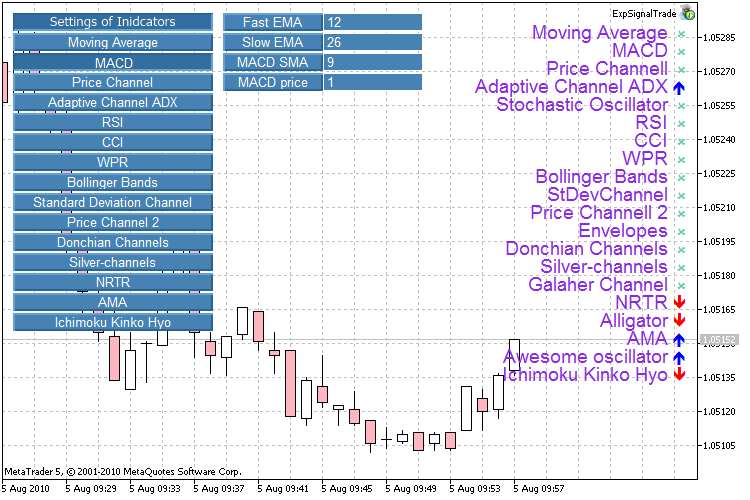We are not going to discuss the implementation of the graphical interface; you can find the information about it in the Creating Active Control Panels in MQL5 for Trading article.

To change the settings of the indicators through our graphical interface, let's improve our SignalTrade.mqh library and name it SignalTradeExp.mqh.

First of all, we need additional variables for storing the settings of indicators.

```//--- input parameters Moving Average
int                periodma1=8;
int                periodma2=16;
ENUM_MA_METHOD     MAmethod=MODE_SMA;
ENUM_APPLIED_PRICE MAprice=PRICE_CLOSE;
//--- input parameters MACD
int                FastMACD=12;
int                SlowMACD=26;
int                MACDSMA=9;
ENUM_APPLIED_PRICE MACDprice=PRICE_CLOSE;
//--- input parameters Price Channel
int                PCPeriod=22;
//--- input parameters Stochastic Oscillator
int                SOPeriodK=5;
int                SOPeriodD=3;
int                SOslowing=3;
ENUM_MA_METHOD     SOmethod=MODE_SMA;
ENUM_STO_PRICE     SOpricefield=STO_LOWHIGH;
//--- input parameters RSI
int                RSIPeriod=14;
ENUM_APPLIED_PRICE RSIprice=PRICE_CLOSE;
//--- input parameters CCI
int                CCIPeriod=14;
ENUM_APPLIED_PRICE CCIprice=PRICE_TYPICAL;
//--- input parameters WPR
int                WPRPeriod=14;
//--- input parameters Bollinger Bands
int                BBPeriod=20;
double             BBdeviation=2.0;
ENUM_APPLIED_PRICE BBprice=PRICE_CLOSE;
//--- input parameters Standard Deviation Channel
int                SDCPeriod=14;
double             SDCdeviation=2.0;
ENUM_APPLIED_PRICE SDCprice=PRICE_CLOSE;
ENUM_MA_METHOD     SDCmethod=MODE_SMA;
//--- input parameters Price Channel 2
int                PC2Period=22;
//--- input parameters Envelopes
int                ENVPeriod=14;
double             ENVdeviation=0.1;
ENUM_APPLIED_PRICE ENVprice=PRICE_CLOSE;
ENUM_MA_METHOD     ENVmethod=MODE_SMA;
//--- input parameters Donchian Channels
int                DCPeriod=24;
int                DCExtremes=3;
int                DCMargins=-2;
//--- input parameters Silver-channels
int                SCPeriod=26;
double             SCSilvCh=38.2;
double             SCSkyCh=23.6;
double             SCFutCh=61.8;
//--- input parameters NRTR
int                NRTRPeriod   =  40;
double             NRTRK        =  2.0;
//--- input parameters Alligator
int                ALjawperiod=13;
int                ALteethperiod=8;
int                ALlipsperiod=5;
ENUM_MA_METHOD     ALmethod=MODE_SMMA;
ENUM_APPLIED_PRICE ALprice=PRICE_MEDIAN;
//--- input parameters AMA
int                AMAperiod=9;
int                AMAfastperiod=2;
int                AMAslowperiod=30;
ENUM_APPLIED_PRICE AMAprice=PRICE_CLOSE;
//--- input parameters Ichimoku Kinko Hyo
int                IKHtenkansen=9;
int                IKHkijunsen=26;
int                IKHsenkouspanb=52;```

Replace the constant values of the indicators with the variables. Other things should be left unchanged.

`h_ma1=iMA(Symbol(),Period(),periodma1,0,MAmethod,MAprice);`

A significant point is the economical usage of the computer memory; when changing the settings it is necessary to unload the copy of indicator with old settings and load a new one. It can be done using the following function:

```bool  IndicatorRelease(
int       indicator_handle,     // indicator handle
);
```
```   if(id==CHARTEVENT_OBJECT_ENDEDIT && sparam=="PIPSetEditMA2")
{
periodma2=(int)ObjectGetString(0,"PIPSetEditMA2",OBJPROP_TEXT);
ObjectSetString(0,"PIPSetEditMA2",OBJPROP_TEXT,(string)periodma2);
//--- unload old copy of the indicator
IndicatorRelease(h_ma2);
//--- create new copy of the indicator
h_ma2=iMA(Symbol(),Period(),periodma2,0,MAmethod,MAprice);
ChartRedraw();
}```

### Conclusion

Thus, we learned how to read information from indicators and how to pass it to Expert Advisor. In such a manner, you can obtain signals from any indicator.

Note

• File of the SignalTrade.mq5, AdaptiveChannelADX.mq5, Donchian Channels.mq5, NRTR.mq5, Price Channel.mq5, PriceChannelGalaher.mq5, Silver-channels.mq5, StandardDeviationChannel.mq5 indicators should be copied to the ...\MQL5\Indicators folder.
• The SignalTrade.mqh and SignalTradeExp.mqh include files should be copied to the ...\MQL5\Include folder.
• The LibFunctions.mq5 library of functions should be copied to the ...\MQL5\Libraries folder.

Translated from Russian by MetaQuotes Software Corp.
Original article: https://www.mql5.com/ru/articles/130

Attached files |
indicators.zip (8.9 KB)
Last comments | Go to discussion (8)| 19 Sep 2010 at 22:02

The code might be of good value but some of the indicators are redundant:

BB and Standard Deviation Channel - same thing;

Price , Donchian and Gallagher  Channels - same thing;

Anyway, thanks for the article...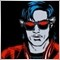| 19 Aug 2012 at 21:53

These are really useful for learning, thanks.| 8 Oct 2013 at 03:56
Good for beginners like me, very  useful. thanks.| 14 Dec 2013 at 07:26

Hello and thank you so much,

I was looking for such advisor for so long, my question is that how can I change the font size? sometimes it is too big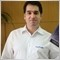| 26 Jun 2014 at 16:58
ravaji:

Hello and thank you so much,

I was looking for such advisor for so long, my question is that how can I change the font size? sometimes it is too big

Not clear why the bars are not equivalent.

I understand, these shoud be like this ...

Is this an error ?

```//--- check the condition and set a value for the sig
if(ma1_buffer<ma2_buffer && ma1_buffer>ma2_buffer)
sig=1;
else if(ma1_buffer>ma2_buffer && ma1_buffer<ma2_buffer)
sig=-1;
else sig=0;

return(sig);```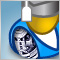The Prototype of Trade Robot

This article summarizes and systematizes the principles of creating algorithms and elements of trading systems. The article considers designing of expert algorithm. As an example the CExpertAdvisor class is considered, which can be used for quick and easy development of trading systems.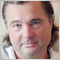Interview with Leonid Velichkovsky: "The Biggest Myth about Neural Networks is Super-Profitability" (ATC 2010)

The hero of our interview Leonid Velichkovski (LeoV) has already participated in Automated Trading Championships. In 2008, his multicurrency neural network was like a bright flash in the sky, earning \$110,000 in a certain moment, but eventually fell victim to its own aggressive money management. Two years ago, in his interview Leonid share his own trading experience and told us about the features of his Expert Advisor. On the eve of the ATC 2010, Leonid talks about the most common myths and misconceptions associated with neural networks.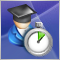How to Quickly Create an Expert Advisor for Automated Trading Championship 2010

In order to develop an expert to participate in Automated Trading Championship 2010, let's use a template of ready expert advisor. Even novice MQL5 programmer will be capable of this task, because for your strategies the basic classes, functions, templates are already developed. It's enough to write a minimal amount of code to implement your trading idea.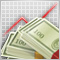Using the TesterWithdrawal() Function for Modeling the Withdrawals of Profit

This article describes the usage of the TesterWithDrawal() function for estimating risks in trade systems which imply the withdrawing of a certain part of assets during their operation. In addition, it describes the effect of this function on the algorithm of calculation of the drawdown of equity in the strategy tester. This function is useful when optimizing parameter of your Expert Advisors.